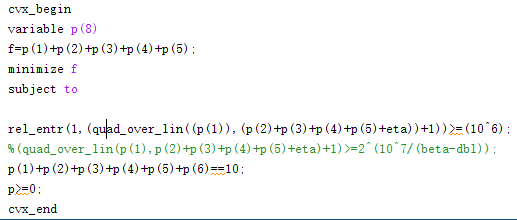Hello,i have a problem.my optimization problem is ‘minimize P P=p1+p2+p3+p4+p5’,
One of the constraints is
%（log2（1+p1/(p2+p3+p4+p5+eta)））>=10^6)
but this is what happens when I run the code
Disciplined convex programming error:
Illegal operation: rel_entr( {positive constant}, {convex} ).

I can’t solve this problem ,I hope you can give me some advice.Thank you very much!!!

`log1+(x/(x+y))`, where `x` and `y` are both variables, is neither convex nor concave.

However, if
`log2（1+p1/(p2+p3+p4+p5+eta)））>=10^6)`
is actually the desired constraint, you can exponentiate (base 2) both sides, thereby eliminating the `log`, then multiply out the denominator, which will result in an affine inequality, which CVX will accept. However, the `2^(10^6)` coefficient will be a total numerical disaster. Maybe you need to think through your model and input data.

But then why do you have `quad_over_lin` some places/ Conclusion, I don’t even know what your desired model is. But you need to make sure it is convex.

if you do have
`log2(quad_over_lin(...)) >= 10^6`
you could exponentiate out the `log2`, but then you would have `{convex} >= constant`, which is going in the wrong direction to be a convex constraint.